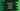# JavaScript String search method explanation with example## JavaScript String search method:

The search method is defined in String object of JavaScript. Strings are used to represent a sequence of characters. search() method is used to search for a match in a string.

We can pass one regular expression or a string to search in a string by using the search() method.

In this post, we will learn how to use the search() method with example.

The search method is defined as like below:

``search(regular_expression)``

It takes one regular expression as the parameter. If we pass any non-regular expression object, it converts that to a regular expression by using new RegExp(re), where re is the non-regular expression object.

### Return value:

This method returns the index of the first occurrance of the substring in the string if it is found. Else, it returns -1.

The index starts at 0, i.e. the index of the first character is 0, index of the second character is 1, etc.

Let’s try search() to search for a sub-string in a string. The below example searches for a string using search() and it prints the result:

``````const givenStr = 'The quick brown fox jumps over the lazy dog';

const strArr = ['dog', 'Dog', 'Hello'];

strArr.forEach(s => {
console.log(`\${s} found at index: \${givenStr.search(s)}`);
});``````

Here,

• givenStr is the string to search for the words.
• strArr is an array with words we are searching in givenStr.
• By using a forEach loop, it is iterating through the words of the string array and for each word it finds, it checks if it is in the string givenStr or not.

If you run the above program, it will print the below output:

``````dog found at index: 40
Dog found at index: -1
Hello found at index: -1``````

### Example with a regular expression:

We can also use a regular expression to match for a sub-string in a string. For example:

``````const givenStr = 'The quick brown fox jumps over the 2lazy dog';

const regex = /[1-9]/;

console.log(givenStr.search(regex));``````

It will print the index of 2, i.e. 35.

### Check if a string is found and print a message:

We can check the return value of search and if it returns -1, we can say that the sub-string is not found in the string. For example:

``````const givenStr = 'The quick brown fox jumps over the lazy dog';

const str = 'fox';

if(givenStr.search(str) == -1){
``fox is found in 'The quick brown fox jumps over the lazy dog'``